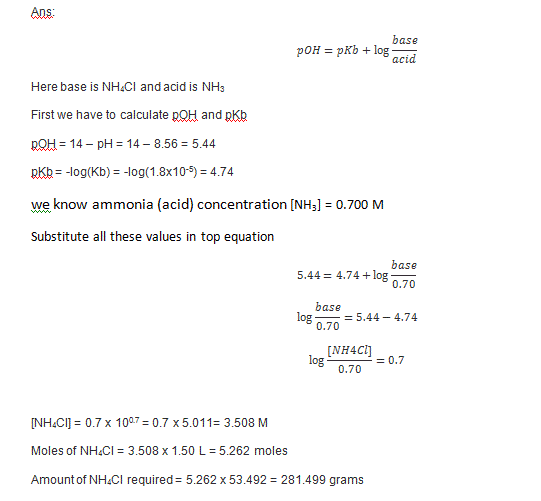Question

# How many grams of dry NH4Cl need to be added to 1.50 L of a 0.700...

How many grams of dry NH4Cl need to be added to 1.50 L of a 0.700 M solution of ammonia, NH3, to prepare a buffer solution that has a pH of 8.56? Kb for ammonia is 1.8×10−5.#### Earn Coins

Coins can be redeemed for fabulous gifts.

Similar Homework Help Questions
• ### How many grams of dry NH4Cl need to be added to 2.00 L of a 0.700...

How many grams of dry NH4Cl need to be added to 2.00 L of a 0.700 M solution of ammonia, NH3, to prepare a buffer solution that has a pH of 9.00? Kb for ammonia is 1.8×10−5. Express your answer with the appropriate units.

• ### How many grams of dry NH4Cl need to be added to 2.50 L of a 0.700...

How many grams of dry NH4Cl need to be added to 2.50 L of a 0.700 M solution of ammonia, NH3, to prepare a buffer solution that has a pH of 8.62? Kbfor ammonia is 1.8×10−5. Express your answer with the appropriate units.

• ### How many grams of dry NH4Cl need to be added to 1.50 L of a 0.500 M solution of ammonia, NH3,to prepare a buffer solutio...

How many grams of dry NH4Cl need to be added to 1.50 L of a 0.500 M solution of ammonia, NH3,to prepare a buffer solution that has a pH of 8.79? Kb for ammonia is 1.8*10^-5.

• ### How many grams of dry NH4Cl need to be added to 1.90 L of a 0.800...

How many grams of dry NH4Cl need to be added to 1.90 L of a 0.800 M solution of ammonia, NH3, to prepare a buffer solution that has a pH of 8.74? Kb for ammonia is 1.8×10^-5.

• ### How many grams of dry NH4Cl need to be added to 1.70 L of a 0.500...

How many grams of dry NH4Cl need to be added to 1.70 L of a 0.500 M solution of ammonia, NH3, to prepare a buffer solution that has a pH of 8.51? Kb for ammonia is 1.8 x 10^-5.

• ### How many grams of dry NH4Cl need to be added to 1.90 L of a 0.800...

How many grams of dry NH4Cl need to be added to 1.90 L of a 0.800 M solution of ammonia, NH3, to prepare a buffer solution that has a pH of 8.74? Kb for ammonia is 1.8×10^−5. Express your answer with the appropriate units.

• ### 4B. How many grams of dry NH4Cl need to be added to 1.80 L of a...

4B. How many grams of dry NH4Cl need to be added to 1.80 L of a 0.100 M solution of ammonia, NH3, to prepare a buffer solution that has a pH of 8.93? Kb for ammonia is 1.8×10^−5. Express your answer with the appropriate units.

• ### How many grams of dry NH4Cl need to be added to 2.50 L of a 0.500 M solution of ammonia, NH3, to prepare a buffer soluti...

How many grams of dry NH4Cl need to be added to 2.50 L of a 0.500 M solution of ammonia, NH3, to prepare a buffer solution that has a pH of 8.74? Kb for ammonia is 1.8*10^-5.

• ### How many grams of dry NH4Cl need to be added to 1.70 L of a 0.100 M solution of ammonia, NH3, to prepare a buffer solu...

How many grams of dry NH4Cl need to be added to 1.70 L of a 0.100 M solution of ammonia, NH3, to prepare a buffer solution that has a pH of 8.62? Kb for ammonia is 1.8×10−5.

• ### How many grams of dry NH4Cl need to be added to 2.50 L of a 0.400 M solution of ammonia, NH3, to prepare a buffer solu...

How many grams of dry NH4Cl need to be added to 2.50 L of a 0.400 M solution of ammonia, NH3, to prepare a buffer solution that has a pH of 8.67? Kb for ammonia is 1.8×10−5.